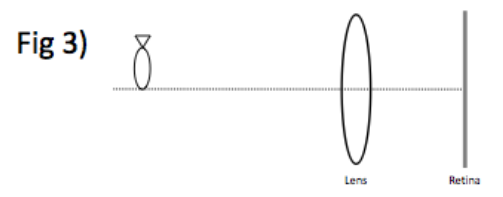# Problem: Draw a light ray diagram for a human eye submerged in water (index of refraction nw = 1.33) where the lens is unable to bring the object into focus. (see figure 3)

###### FREE Expert Solution

Focal length:

$\overline{)\frac{\mathbf{1}}{\mathbf{f}}{\mathbf{=}}{\mathbf{\left(}}{{\mathbf{\eta }}}_{\mathbf{e}\mathbf{y}\mathbf{e}}{\mathbf{-}}{{\mathbf{\eta }}}_{\mathbf{i}\mathbf{n}\mathbf{c}\mathbf{i}\mathbf{d}\mathbf{e}\mathbf{n}\mathbf{t}}{\mathbf{\right)}}{\mathbf{\left(}}\frac{\mathbf{1}}{{\mathbf{R}}_{\mathbf{1}}}{\mathbf{-}}\frac{\mathbf{1}}{{\mathbf{R}}_{\mathbf{2}}}{\mathbf{\right)}}}$

Increasing the η for 1 in air to 1.33 in water reduces the factor (ηeye - ηincident). Thus, (1/f) decreases. The power of the lens will decrease. Therefore, the ability to converge light decreases.

The image is formed past the Retina.

94% (207 ratings)###### Problem Details

Draw a light ray diagram for a human eye submerged in water (index of refraction nw = 1.33) where the lens is unable to bring the object into focus. (see figure 3)